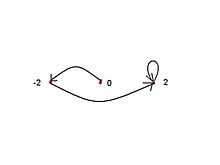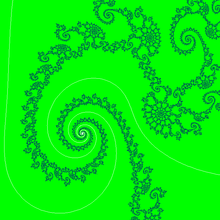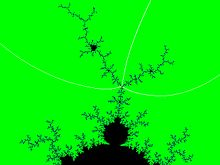# Misiurewicz point

﻿
Misiurewicz point

A Misiurewicz point is a parameter in the Mandelbrot set (the parameter space of quadratic polynomials) for which the critical point is strictly preperiodic (i.e., it becomes periodic after finitely many iterations but is not periodic itself). By analogy, the term Misiurewicz point is also used for parameters in a Multibrot set where the unique critical point is strictly preperiodic. (This term makes less sense for maps in greater generality that have more than one (free) critical point because some critical points might be periodic and others not.)

## Mathematical notation

A parameter c is a Misiurewicz point$M_{k,n} \,$ if it satisfies the equations$f_c^{(k)}(z_{cr}) = f_c^{(k+n)}(z_{cr}) \,$

and$f_c^{(k-1)}(z_{cr}) \neq f_c^{(k+n-1)}(z_{cr})\,$

so :$M_{k,n} = c : f_c^{(k)}(z_{cr}) = f_c^{(k+n)}(z_{cr}) \,$

where :

•$z_{cr} \,$ is a critical point of$f_c\,$,
•$k\,$ and$n\,$ are positive integers,

and$f_c^{k}$ denotes the k-th iterate of fc.

## Name

Misiurewicz points are named after the Polish-American mathematician Michał Misiurewicz.

Note that the term "Misiurewicz point" is used ambiguously: Misiurewicz originally investigated maps in which all critical points were non-recurrent (that is, there is a neighborhood of every critical point that is not visited by the orbit of this critical point), and this meaning is firmly established in the context of dynamics of iterated interval maps. The case that for a quadratic polynomial the unique critical point is strictly preperiodic is only a very special case; in this restricted sense (as described above) this term is used in complex dynamics; a more appropriate term would be Misiurewicz-Thurston points (after William Thurston who investigated postcritically finite rational maps).

A complex quadratic polynomial has only one critical point. By a suitable conjugation any quadratic polynomial can be transformed into a map of the form$P_c(z)=z^2+c \,$ which has a single critical point at$z = 0\,$. The Misiurewicz points of this family of maps are roots of the equations$P_c^{(k)}(0) = P_c^{(k+n)}(0)$,

(subject to the condition that the critical point is not periodic), where :

• k is the pre-period
• n is the period
•$P_c^{(n)} = P_c ( P_c^{(n-1)})\,$ denotes the n-fold composition of$P_c(z)=z^2+c\,$ with itself i.e. the nth iteration of$P_c\,$.

For example, the Misiurewicz points with k=2 and n=1, denoted by M2,1, are roots of$P_c^{(2)}(0) = P_c^{(3)}(0)$$\Rightarrow c^2+c=(c^2+c)^2+c$$\Rightarrow c^4+2c^3=0$.

The root c=0 is not a Misiurewicz point because the critical point is a fixed point when c=0, and so is periodic rather than pre-periodic. This leaves a single Misiurewicz point M2,1 at c = −2.

## Properties of Misiurewicz points of complex quadratic mapping

Misiurewicz points belong to the boundary of the Mandelbrot set. Misiurewicz points are dense in the boundary of the Mandelbrot set.

If$c\,$ is a Misiurewicz point, then the associated filled Julia set is equal to the Julia set, and means the filled Julia set has no interior.

If$c\,$ is a Misiurewicz point, then in the corresponding Julia set all periodic cycles are repelling (in particular the cycle that the critical orbit falls onto).

Mandelbrot set and Julia set$J_c\,$ are locally asymptotically similar around Misiurewicz points. Mandelbrot set is self-similar around Misiurewicz points 

### Types

Misiurewicz points can be classified according to number of external rays that land on them :

• branch points ( = points that disconnect the Mandelbrot set into at least three components.) with 3 or more external arguments ( angles )
• non-branch points with exactly 2 external arguments ( = interior points of arcs within the Mandelbrot set) : these points are less conspicuous and thus not so easily to find on pictures.
• end points with 1 external argument

According to the Branch Theorem of the Mandelbrot set, all branch points of the Mandelbrot set are Misiurewicz points (plus, in a combinatorial sense, hyperbolic components represented by their centers).

Many (actually, most) Misiurewicz parameters in the Mandelbrot set look like centers of spirals'. The explanation for this is the following: at a Misiurewicz parameter, the critical value jumps onto a repelling periodic cycle after finitely many iterations; at each point on the cycle, the Julia set is asymptotically self-similar by a complex multiplication by the derivative of this cycle. If the derivative is non-real, then this implies that the Julia set, near the periodic cycle, has a spiral structure. A similar spiral structure thus occurs in the Julia set near the critical value and, by Tan Lei's aforementioned theorem, also in the Mandelbrot set near any Misiurewicz parameter for which the repelling orbit has non-real multiplier. Depending on the value of the multiplier, the spiral shape can seem more or less pronounced. The number of the arms at the spiral equals the number of branches at the Misiurewicz parameter, and this equals the number of branches at the critical value in the Julia set. (Even the principal Misiurewicz point in the 1/3-limb', at the end of the parameter rays at angles 9/56, 11/56, and 15/56, turns out to be asymptotically a spiral, with infinitely many turns, even though this is hard to see without maginification.)

### External arguments

External arguments of Misiurewicz points, measured in turns are :

• rational numbers
• proper fraction with even denominator
• dyadic fractions with denominator$= 2^b\,$ and finite ( terminating ) expansion , like :$\frac{1}{2}_{10} = 0.5_{10} = 0.1_2 \,$
• fraction with denominator$= a*2^b\,$ and repeating expansion like :$\frac{1}{6}_{10} = \frac{1}{2*3}_{10}= 0,16666..._{10} = 0.0(01)..._2\,$.

where :

a and b are positive integers and b is odd ,

subscript number shows base of numeral system.

## Examples of Misiurewicz points of complex quadratic mapping

### End pointsOrbit of critical point$z = 0\,$ under$f_{-2}\,$$c =M_{2,1}\,$

Point$c = M_{2,2} = i \,$  :

• is a tip of the filament
• Its critical orbits is$\{0, i, i-1, -i, i-1, -i...\} \,$ 

Point$c = M_{2,1} = -2\,$

• is the end-point of main antenna of Mandelbrot set 
• Its critical orbits is$\{ 0 , -2, 2, 2, 2, ... \} \,$ 
• Symbolic sequence = C L R R R ...
• preperiod is 2 and period 1

Notice that it is z-plane (dynamical plane) not c-plane (parameter plane) and point$z = -2\,$ is not the same point as$c = -2\,$.

Point$c = -2 =M_{2,1}\,$ is landing point of only one external ray ( parameter ray) of angle 1/2 .

### Non-Branch points$c =M_{23,3}\,$

Point$c= -0.77568377+0.13646737*i \,$ is near a Misiurewicz point$M_{23,2}\,$. It is

• a center of a two-arms spiral
• a landing point of 2 external rays with angles :$\frac{8388611}{25165824}\,$ and$\frac{8388613}{25165824}\,$ where denominator is$3*2^{23}\,$
• preperiodic point with preperiod$k = 23\,$ and period$n = 2\,$

Point$c = -1.54368901269109 \,$ is near a Misiurewicz point$M_{3,2}\,$ ,

• which is landing point for pair of rays :$\frac{5}{12}\,$ ,$\frac{7}{12}\,$
• has preperiod$k = 3\,$ and period$n = 2\,$

### Branch points$c =M_{4,1}\,$

Point$c = -0.1010... +0.9562...*i =M_{4,1}\,$ is a principal Misiurewicz point of the 1/3 limb. It has 3 external rays 9/56, 11/56 and 15/56.

2. ^ Wellington de Melo, Sebastian van Strien, "One-dimensional dynamics". Monograph, Springer Verlag (1991)
3. ^ a b c Adrien Douady, John Hubbard, "Etude dynamique des polynômes complexes", prépublications mathématiques d'Orsay, 1982/1984
4. ^ a b c Dierk Schleicher, "On Fibers and Local Connectivity of Mandelbrot and Multibrot Sets", in: M. Lapidus, M. van Frankenhuysen (eds): Fractal Geometry and Applications: A Jubilee of Benoît Mandelbrot. Proceedings of Symposia in Pure Mathematics 72, American Mathematical Society (2004), 477–507 or online paper from arXiv.org
5. ^ Lei.pdf Tan Lei, "Similarity between the Mandelbrot set and Julia Sets", Communications in Mathematical Physics 134 (1990), pp. 587-617.
6. ^ The boundary of the Mandelbrot set by Michael Frame, Benoit Mandelbrot, and Nial Neger
7. ^ BINARY DECIMAL NUMBERS AND DECIMAL NUMBERS OTHER THAN BASE TEN by Thomas Kim-wai YEUNG and Eric Kin-keung POON
8. ^ Tip of the filaments by Robert P. Munafo
9. ^ a b Preperiodic (Misiurewicz) points in the Mandelbrot se by Evgeny Demidov
10. ^ tip of main antennae by Robert P. Munafo

Wikimedia Foundation. 2010.

### Look at other dictionaries:

• Michał Misiurewicz — Born November 9, 1948 (1948 11 09) (age 63) Warsaw, Poland Residence …   Wikipedia

• Mandelbrot set — Initial image of a Mandelbrot set zoom sequence with a continuously coloured environment …   Wikipedia

• Ensemble de Mandelbrot — L ensemble de Mandelbrot (en noir) L ensemble de Mandelbrot est une fractale définie comme l ensemble des points c du plan complexe pour lesquels la suite définie par récurrence par  …   Wikipédia en Français

• Complex quadratic polynomial — A complex quadratic polynomial is a quadratic polynomial whose coefficients are complex numbers. Contents 1 Forms 2 Conjugation 2.1 Between forms 2.2 With doubling map …   Wikipedia

• Julia set — In complex dynamics, the Julia set J(f), [Note that in other areas of mathematics the notation J(f), can also represent the Jacobian matrix of a real valued mapping f, between smooth manifolds.] of a holomorphic function f, informally consists of …   Wikipedia

• Filled Julia set — The filled in Julia set K(f c) of a polynomial f c is defined as the set of all points z, of dynamical plane that have bounded orbit with respect to f c K(f c) overset{underset{mathrm{def{{=} { z in mathbb{C} : f^{(k)} c (z) ot o infty as k o… …   Wikipedia

• External ray — In complex analysis, particularly in complex dynamics and geometric function theory, external rays are associated to a compact, full, connected subset K, of the complex plane as the images of radial rays under the Riemann map of the complement of …   Wikipedia

• Self-similarity — NOTOC [ thumb|right|250px|A Koch curve has an infinitely repeating self similarity when it is magnified.] In mathematics, a self similar object is exactly or approximately similar to a part of itself (i.e. the whole has the same shape as one or… …   Wikipedia

• List of mathematics articles (M) — NOTOC M M estimator M group M matrix M separation M set M. C. Escher s legacy M. Riesz extension theorem M/M/1 model Maass wave form Mac Lane s planarity criterion Macaulay brackets Macbeath surface MacCormack method Macdonald polynomial Machin… …   Wikipedia

• SYSTÈMES DYNAMIQUES DIFFÉRENTIABLES — Sans doute née avec le mémoire que Poincaré écrivit en 1881 «sur les courbes définies par des équations différentielles», où l’étude quantitative (analytique) locale des équations différentielles dans le champ complexe est remplacée par leur… …   Encyclopédie Universelle